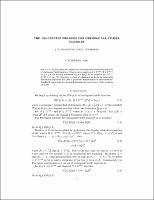## The Procrustes Problem for Orthogonal Stiefel Matrices##### Abstract
(This abstract contains mathematical symbols that may not reproduce wellin ASCII text.) In this paper we consider the Procrustes problem on the manifold of orthogonal Stiefel matrices. That is, given matrices A epsilon R(m*k), B epsilon R(m*p), m greater tahn or equal to p greater than or equal to k, we seek the minimum of ||A - BQ||2 for all matrices Q epsilon R(p*k), QTQ = I(k*k). We introduce a class of relaxation methods for generating minimizing sequences and offer a geometric interpretation of these methods. Results of numerical experiments illustrating the convergence of the methods are given.
1996-08
##### Publisher
Cornell University
theory center
##### Previously Published As
http://techreports.library.cornell.edu:8081/Dienst/UI/1.0/Display/cul.tc/96-255
technical report